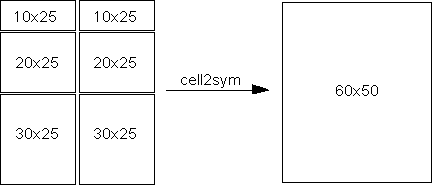# cell2sym

Convert cell array to symbolic array

## Syntax

``S = cell2sym(C)``
``S = cell2sym(C,flag)``

## Description

example

````S = cell2sym(C)` converts a cell array `C` to a symbolic array `S`. The elements of `C` must be convertible to symbolic objects.If each element of the input cell array `C` is a scalar, then `size(S) = size(C)`, and ```S(k) = sym(C(k))``` for all indices `k`. If the cell array `C` contains nonscalar elements, then the contents of `C` must support concatenation into an N-dimensional rectangle. Otherwise, the results are undefined. For example, the contents of cells in the same column must have the same number of columns. However, they do not need to have the same number of rows. See figure.```

example

````S = cell2sym(C,flag)` uses the technique specified by flag for converting floating-point numbers to symbolic numbers.```

## Examples

### Convert Cell Array of Scalars

Convert a cell array of only scalar elements to a symbolic array.

Create a cell array of scalar elements.

`C = {'x','y','z'; 1 2 3}`
```C = 2×3 cell array {'x'} {'y'} {'z'} {} {} {}```

Convert this cell array to a symbolic array.

`S = cell2sym(C)`
```S = [ x, y, z] [ 1, 2, 3]```

`cell2sym` does not create symbolic variables `x`, `y`, and `z` in the MATLAB® workspace. To access an element of `S`, use parentheses.

`S(1,1)`
```ans = x```

### Convert Cell Array Containing Nonscalar Elements

Convert a cell array whose elements are scalars, vectors, and matrices into a symbolic array. Such conversion is possible only if the contents of the cell array can be concatenated into an N-dimensional rectangle.

Create a cell array, the elements of which are a scalar, a row vector, a column vector, and a matrix.

`C = {'x' [2 3 4]; ['y'; sym(9)] [6 7 8; 10 11 12]}`
```C = 2×2 cell array {'x' } {1×3 double} {2×1 sym} {2×3 double}```

Convert this cell array to a symbolic array.

`S = cell2sym(C)`
```S = [ x, 2, 3, 4] [ y, 6, 7, 8] [ 9, 10, 11, 12]```

### Choose Conversion Technique for Floating-Point Values

When converting a cell array containing floating-point numbers, you can explicitly specify the conversion technique.

Create a cell array `pi` with two elements: the double-precision value of the constant `pi` and the exact value `pi`.

`C = {pi, sym(pi)}`
```C = 1×2 cell array {[3.1416]} {1×1 sym}```

Convert this cell array to a symbolic array. By default, `cell2sym` uses the rational conversion mode. Thus, results returned by `cell2sym` without a flag are the same as results returned by `cell2sym` with the flag `'r'`.

`S = cell2sym(C)`
```S = [ pi, pi]```
`S = cell2sym(C,'r')`
```S = [ pi, pi]```

Convert the same cell array to a symbolic array using the flags `'d'`, `'e'`, and `'f'`. See the Input Arguments section for the details about conversion techniques.

`S = cell2sym(C,'d')`
```S = [ 3.1415926535897931159979634685442, pi]```
`S = cell2sym(C,'e')`
```S = [ pi - (198*eps)/359, pi]```
`S = cell2sym(C,'f')`
```S = [ 884279719003555/281474976710656, pi]```

## Input Arguments

collapse all

Input cell array, specified as a cell array. The elements of `C` must be convertible to symbolic objects.

Conversion technique, specified as one of the characters listed in this table.

 `'r'` In the rational mode, `cell2sym` converts floating-point numbers obtained by evaluating expressions of the form `p/q`, `p*pi/q`, `sqrt(p)`, `2^q`, and `10^q` for modest sized integers `p` and `q` to the corresponding symbolic form. This approach effectively compensates for the round-off error involved in the original evaluation, but might not represent the floating-point value precisely. If `cell2sym` cannot find simple rational approximation, then it uses the same technique as it would use with the flag `'f'`. `'d'` In the decimal mode, `cell2sym` takes the number of digits from the current setting of `digits`. Conversions with fewer than 16 digits lose some accuracy, while more than 16 digits might not be warranted. For example, `cell2sym({4/3},'d')` with the 10-digit accuracy returns `1.333333333`, while with the 20-digit accuracy it returns `1.3333333333333332593`. The latter does not end in `3`s, but it is an accurate decimal representation of the floating-point number nearest to `4/3`. `'e'` In the estimate error mode, `cell2sym` supplements a result obtained in the rational mode by a term involving the variable `eps`. This term estimates the difference between the theoretical rational expression and its actual floating-point value. For example, `cell2sym({3*pi/4},'e')` returns ```(3*pi)/4 - (103*eps)/249```. `'f'` In the floating-point mode, `cell2sym` represents all values in the form `N*2^e` or `-N*2^e`, where `N >= 0` and `e` are integers. For example, `cell2sym({1/10},'f')` returns `3602879701896397/36028797018963968`. The returned rational value is the exact value of the floating-point number that you convert to a symbolic number.

## Output Arguments

collapse all

Resulting symbolic array, returned as a symbolic array.